# Cubes and Dices - Non Verbal Reasoning

## Cubes and Dices - Non Verbal Reasoning

Q. No 1 to 5: A cube is painted blue on two adjacent surfaces and black on the surfaces opposite to blue surfaces and green on the remaining faces .Now the cube is cut into 216 smaller cubes

of equal size.

1. How many smaller cubes will have no surface painted?

(A) 32 (b) 64 (c) 48 (d) 56

2. How many smaller cube has only one surface painted?

(a) 48 (b) 24 (c) 32 (d) 28

3. How many smaller cubes with two surfaces painted have one face green and one of the adjacent faces red or black?

(a) 36 (b) 42 (c) 32 (d) 48

4. How many smaller cube have three surface painted?

(a) 4 (b) 16 (c) 8 (d) 24

5. How many smaller cubes have less than three surfaces painted?

(a) 44 (b) 32 (c) 64 (d) 144

6. The figure given on the left hand side, in each problem, is folded to form a cube .Choose from amongst the alternatives (a), (b), (c), (d, and the cubes that are similar to the cube

formed.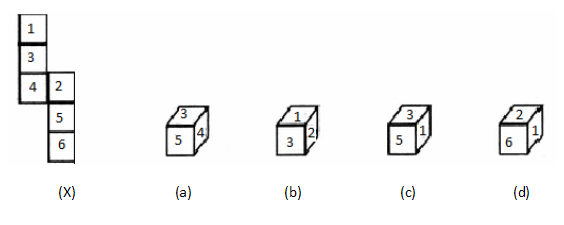7. The figure given on the left hand side, in each problem, is folded to form a cube .Choose from amongst the alternatives (a), (b), (c), (d, and the cubes that are similar to the cube

formed.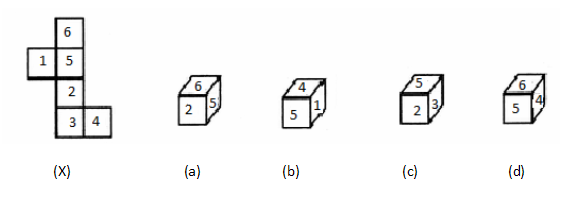8. The figure given on the left hand side, in each problem, is folded to form a cube .Choose from amongst the alternatives (a), (b), (c), (d, and the cubes that are similar to the cube

formed.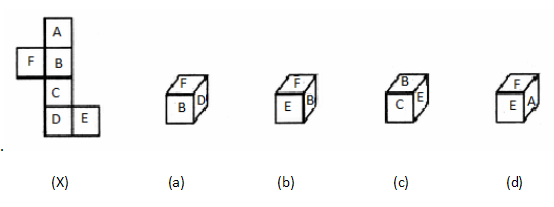9. The figure given on the left hand side, in each problem, is folded to form a cube .Choose from amongst the alternatives (a), (b), (c), (d, and the cubes that are similar to the cube

formed.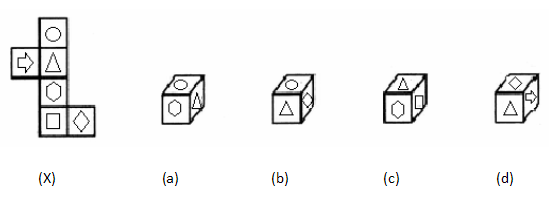10. The figure given on the left hand side, in each problem, is folded to form a cube .Choose from amongst the alternatives (a), (b), (c), (d, and the cubes that are similar to the cube

formed.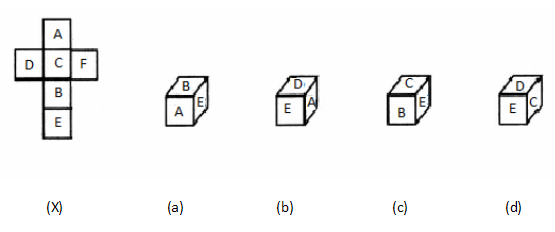Q. No 11 to 15: The outer border of width 1cm of a cube with side 5cm is painted pink on each side and remaining space enclosed by this 1cm path is painted yellow. This cube is now cut into

125 smaller cubes of each side 1cm.The smaller cube so obtained are now separated.

11. How many smaller cubes have one face coloured yellow and an adjacent face pink?

(a) 8 (b) 0 (c) 4 (d) 18

12. How many smaller cubes have three surfaces coloured pink?

(a) 32 (b) 8 (c) 24 (d) 12

13. How many smaller cubes have at least one face coloured?

(a) 78 (b) 84 (c) 98 (d) 96

14. How many smaller cubes have all the surfaces uncoloured?

(a) 27 (b) 36 (c) 49 (d) 18

15. How many smaller cubes have at least two surfaces coloured pink?

(a) 64 (b) 52 (c) 40 (d) 44

16.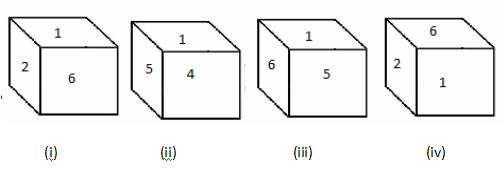Which number is on the opposite surface of number 1?

(a) 5 (b) 3 (c) 4 (d) 2

17.Which letter is opposite to the surface of C?

(a) D (b) A (c) B (d) F

18.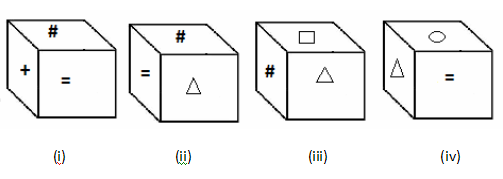Which symbol will come opposite to symbol #?

(a) oval (b) = (c)+ (d) Triangle

19.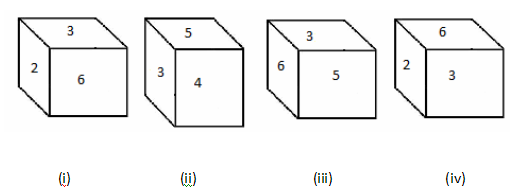Which number is opposite to number 3?

(a) 4 (b) 2 (c) 6 (d) 1